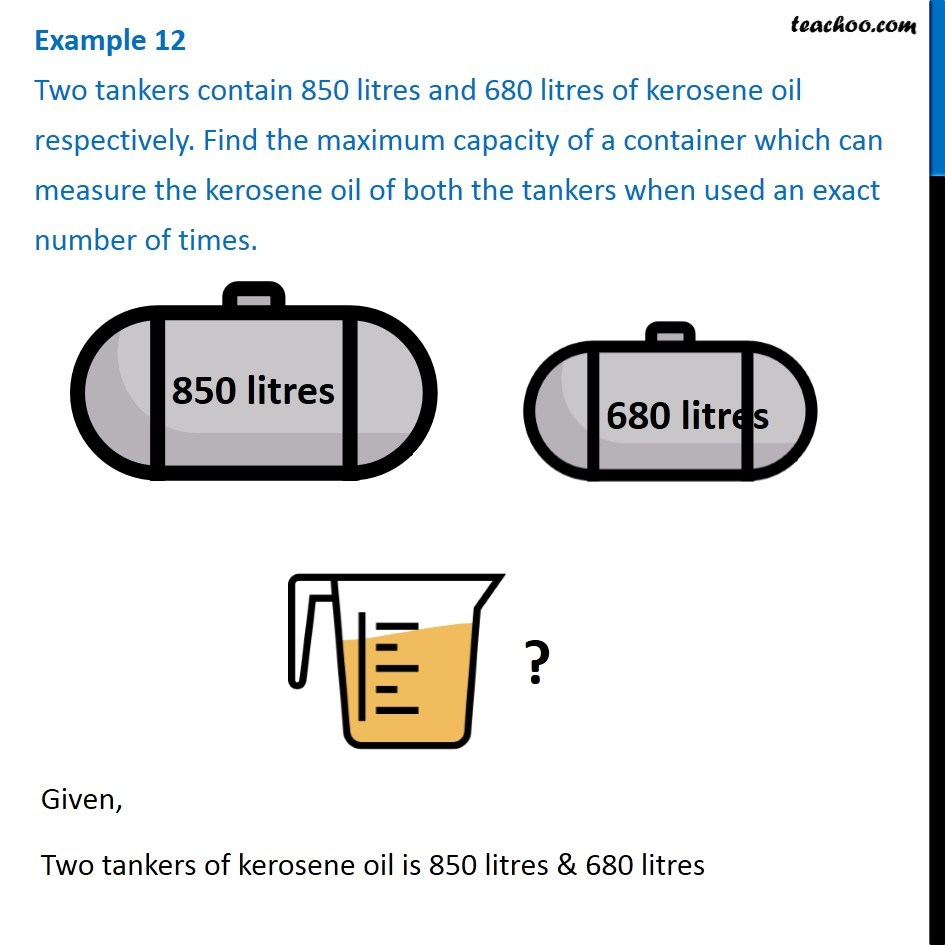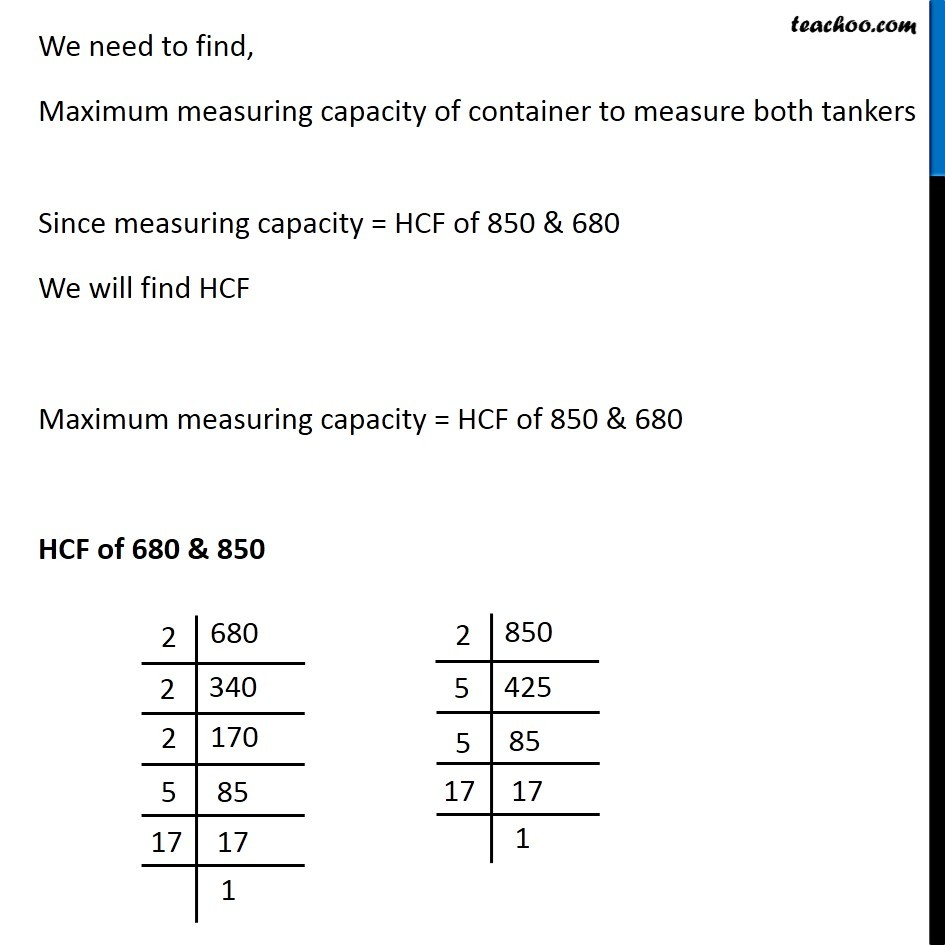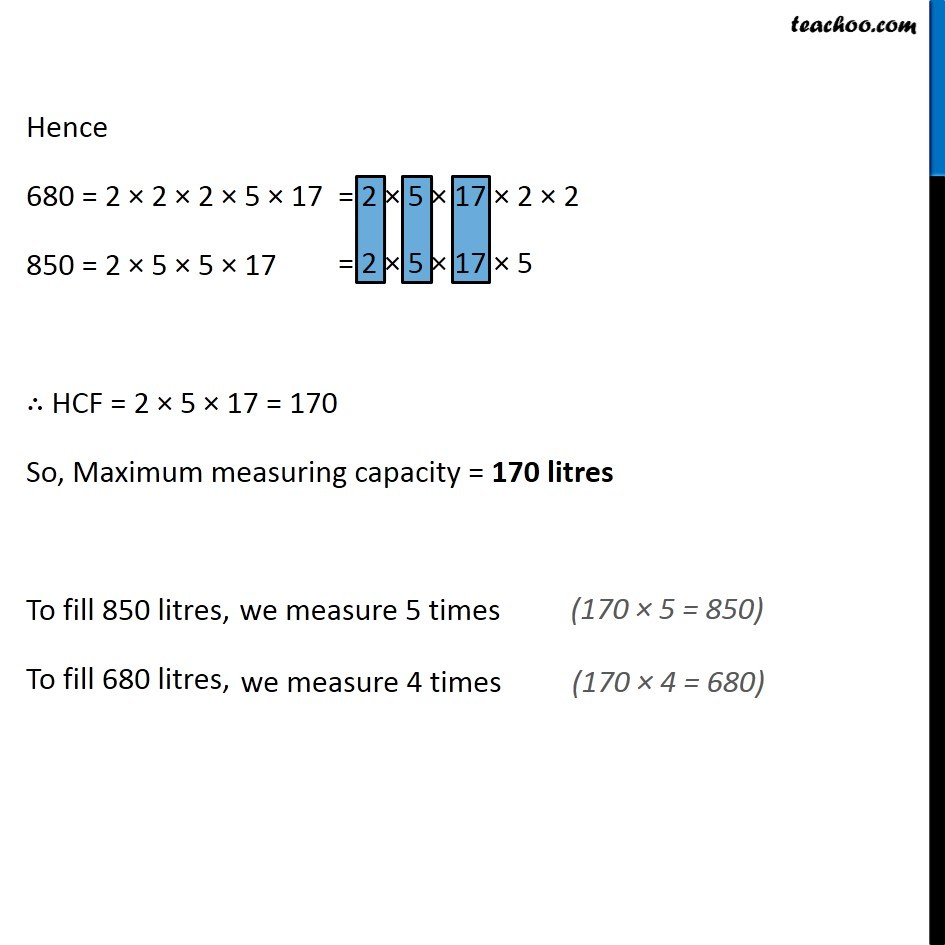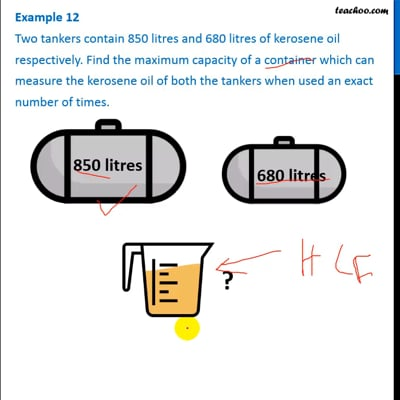Examples

Chapter 3 Class 6 Playing with Numbers
Serial order wiseThis video is only available for Teachoo black users

### Transcript

Example 12 Two tankers contain 850 litres and 680 litres of kerosene oil respectively. Find the maximum capacity of a container which can measure the kerosene oil of both the tankers when used an exact number of times. Given, Two tankers of kerosene oil is 850 litres & 680 litres We need to find, Maximum measuring capacity of container to measure both tankers Since measuring capacity = HCF of 850 & 680 We will find HCF Maximum measuring capacity = HCF of 850 & 680 HCF of 680 & 850 Hence 680 = 2 × 2 × 2 × 5 × 17 850 = 2 × 5 × 5 × 17 ∴ HCF = 2 × 5 × 17 = 170 So, Maximum measuring capacity = 170 litres To fill 850 litres, To fill 680 litres,